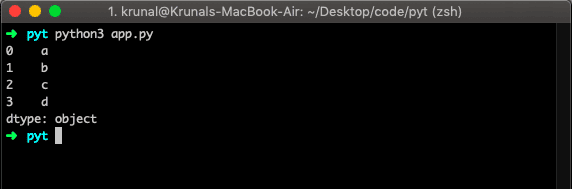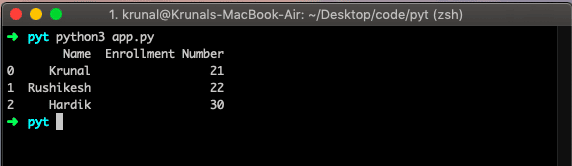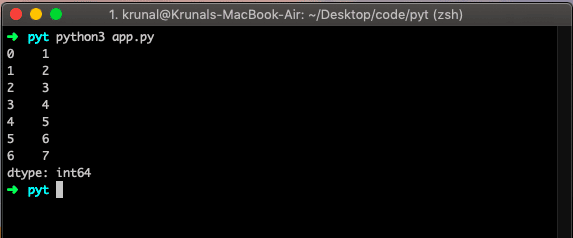# Pandas: Python Data Analysis Library For Machine Learning

Pandas is a software library written for the Python programming language for data manipulation and analysis. Python has been great for data manipulation and preparation but less for data analysis and modeling.

Pandas help fill this gap by enabling you to carry out your entire data analysis workflow in Python without switching to a more domain-specific language like R for data analysis.

Pandas do not implement significant modeling functionality outside of linear and panel regression.

## Key Features of Pandas

The key features of Pandas are the following.

1. Pandas library is a fast and efficient DataFrame object with the default and customized indexing.
2. Pandas library helps load the data into in-memory data objects from different file formats.
3. It has functions that deal with Data alignment and integrated the handling of missing data.
4. Using Pandas, we can reshape and pivot the data sets.
5. It has Label-based slicing, indexing, and subsetting of more massive datasets.
6. Pandas can insert or delete the Columns from the data structure.
7. We can use Pandas for data aggregation and transformations.
8. It gives the High-performance merging and joining of data.
9. Time Series functionality.

## What are Pandas?

Pandas is the Python package providing fast, reliable, flexible, and expressive data structures designed to make working with ‘relational’ or ‘labeled’ data easy and intuitive.

Pandas aim to be the fundamental high-level building block for practical, real-world data modeling and analysis in Python Programming Language.

## Install Pandas on Mac

Install Pandas if you have not installed them previously on your machine.

You can install via PyPI using the following command.

`python3 -m pip install --upgrade pandas`

If you want to upgrade the version, you can use the following command.

`python3 -m pip install --upgrade pandas==0.23.0`

Make sure; you install it with proper permission, such as using sudo if you are on Linux or Mac.

Standard Python distribution does not come with the Pandas module. An alternative way is to install NumPy using a popular Python package installer, pip.

If you have installed a software pack like Anacondathen Pandas have already been installed.

Now, let’s test by the following example.

```# app.py

import pandas as pd
import numpy as np
data = np.array(['a','b','c','d'])
seri = pd.Series(data)
print(seri)
```

Go to the terminal and type the following command to run the file.If you will get the above output, then congrats!!. You have installed the Pandas successfully in your machine.

## Pandas Data Structure

Pandas deals with the following two data structures.

The panel is deprecated and will be removed in a future version.
The recommended way to represent these types of 3-dimensional data is via the Panel via a MultiIndex on a DataFrame.to_frame() method.

### DataFrames in Pandas

DataFrames allow you to store and manipulate the tabular data in rows of observations and columns of variables.

DataFrames in Python are very similar as they come with the Pandas library, and they are defined as two-dimensional labeled data structures with columns of potentially different types.

#### Features of DataFrame

1. Potentially columns are of different types
2. Size – Mutable
3. Labeled axes (rows and columns)
4. Can Perform Arithmetic operations on rows and columns

A pandas DataFrame can be created using the following constructor.

`pandas.DataFrame( data, index, columns, dtype, copy)`

Let’s see the DataFrame example.

```# app.py

import pandas as pd
import numpy as np

data = [['Krunal', 21],['Rushikesh', 22],['Hardik',30]]
df = pd.DataFrame(data, columns=['Name', 'Enrollment Number'])
print(df)```

Now, run the above file and see the output.In the above example, we have taken the data: Name and Enrollment Number. For that data, we have used the NumPy library.

Then, we passed that data to the DataFrame and created a tabular data structure.

### Series in Pandas

Series is the one-dimensional labeled array capable of holding data of any data type like integer, string, float, Python objects, etc. The axis labels are collectively called index.

Labels need not be unique but must be a hashable type. The object supports integer and label-based indexing and provides various methods for performing operations involving the index.

The syntax of Series in Pandas is the following.

`pandas.Series( data, index, dtype, copy)`

Let’s create a primary series.

```# app.py

import pandas as pd

data = [1, 2, 3, 4, 5, 6, 7]
df = pd.Series(data)
print(df)```

Run the file and see the output.That’s it for Pandas library.

This site uses Akismet to reduce spam. Learn how your comment data is processed.